Next: Cartesian Components of a Up: Vectors and Vector Fields Previous: Scalars and Vectors

# Vector Algebra

Suppose that the displacements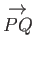and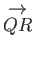, shown in Figure A.2, represent the vectors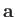and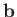, respectively. It can be seen that the result of combining these two displacements is to give the net displacement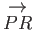. Hence, ifrepresents the vector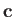then we can write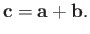(A.1)

This defines vector addition. By completing the parallelogram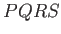, we can also see that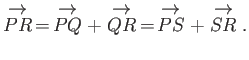(A.2)

However,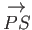has the same length and direction as, and, thus, represents the same vector,. Likewise,and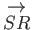both represent the vector. Thus, the previous equation is equivalent to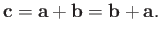(A.3)

We conclude that the addition of vectors is commutative. It can also be shown that the associative law holds: that is,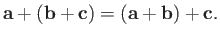(A.4)

The null vector,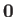, is represented by a displacement of zero length and arbitrary direction. Because the result of combining such a displacement with a finite length displacement is the same as the latter displacement by itself, it follows that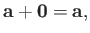(A.5)

whereis a general vector. The negative ofis defined as that vector which has the same magnitude, but acts in the opposite direction, and is denoted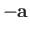. The sum ofandis thus the null vector: that is,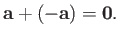(A.6)

We can also define the difference of two vectors,and, as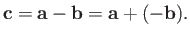(A.7)

This definition of vector subtraction is illustrated in Figure A.3.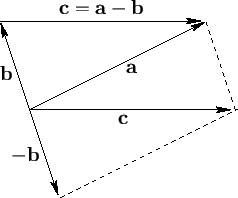If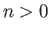is a scalar then the expression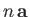denotes a vector whose direction is the same as, and whose magnitude is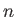times that of. (This definition becomes obvious whenis an integer.) Ifis negative then, because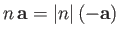, it follows thatis a vector whose magnitude is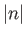times that of, and whose direction is opposite to. These definitions imply that ifandare two scalars then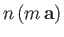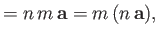(A.8)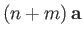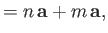(A.9)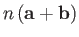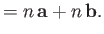(A.10)Next: Cartesian Components of a Up: Vectors and Vector Fields Previous: Scalars and Vectors
Richard Fitzpatrick 2016-03-31